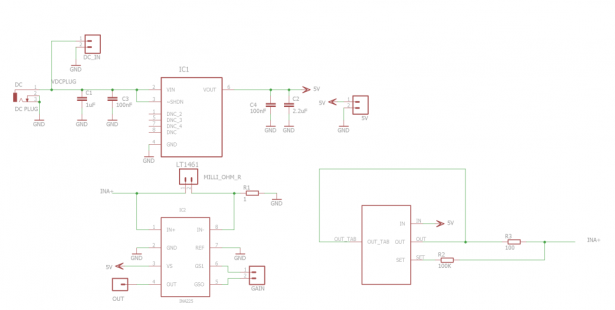# HARDWARE MAKERS

This is an example of a simple and cheap milliohmmeter that can be made by every maker. The core of the circuit are a current source (LT3092) and a current sense (INA225): a costant current flows through the milliohm resistor under test and the voltage at the current sense output gives the value of the resistor (V=R*I).

The milliohmmeter can be used as a stand alone instrument by adding a MCU with at least 10 bit ADC and a LCD display or it can be used togheter with a DMM.Current out from LT3092 can be set between 0.5 mA and 200 mA with 1% accuracy; INA225 gain can be set to 25, 50, 100 or 200 (0.3% accuracy). By setting Iout from current source equal to 10mA (Iout=10*R2/R3 uA) and current sense gain equal to 100, 1Ω = 1V and 1mΩ = 1mV as such the milliohm  resistor value can be easily measured in mV using a DMM, without any kind of conversion. A voltage reference (LT1461) has been added to the circuit in order to have a precise and stable voltage, useful to power both the current source and the current sense as well as the the MCU and its ADC in case we want to have a stand alone milliohmmeter.

Following a simple example of code running on mbed freescale KL-25Z:

 `#include “mbed.h”` `Serial pc(USBTX, USBRX); ``// tx, rx` `float` `VADC=2.922; ``//ADC VOLTAGE REFERENCE (EQUAL TO V SUPPLY)` `//float IO=10.0; //COSTANT CURRENT` `//float GAIN=100.0; //INA225 GAIN` `//BOTH NOT REQUIRED IF SET LIKE THIS (1 Ω = 1 V)` `float` `RO=0.021; ``//RESISTANCE OF CONNECTING CABLES (COMPENSATION)` `float` `STAMPA_R = 0;` `AnalogIn resistor(A0); ``//CONNECTED TO INA225 OUT` `float` `ohm()` `{` `float` `R=0.0;` `float` `RADC=resistor.read();` `R = VADC*RADC-RO; ``//IF IO=10mA AND INA225 GAIN 100 -> (1 Ω = 1 V)` `wait_ms(100);` `return` `R;` `}` `int` `main()` `{` `while` `(1) {` `STAMPA_R= (ohm());` `pc.``printf``(“R = “);` `pc.``printf``(“%1.3f”,STAMPA_R); ``//R IN OHM WITH 3 DECIMALS (1mΩ OF RESOLUTION)` `pc.``printf``(“Ω”);` `pc.``printf``(“\r”);` `wait_ms(500);` `}` `}`

For more detail:  HARDWARE MAKERS